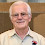## Thursday, November 14, 2013

### Math Stories - Engaging students in thinking about mathematics

In June 2013 I wrote about the Jinx Puzzle where you pick a number and then do several different calculations to that number and the result is 13 no matter what number you started with. I called it the Jinx puzzle for that reason. Why does it work every time? The secret is in the algebra. See below.

1. Choose a number. Call it X
2. Add 11. Now you have X + 11
3. Multiply by 6. Result is: 6X + 66
4. Subtract 3. The result is:  6X - 63
5. Divide by 3. The result is:  2X + 21
6. Add 5. The result is: 2X + 26
7. Divide by 2. The result is: X + 13
8. Subtract the original number that you chose. The result is: X + 13 - X = 13

You are jinxed. Problem solved. Case closed. But what if we were teaching typical 6th graders then a more interesting twist on this story is to not assume the obvious (that it always works) and see whether these students could find a number that foils the Jinx Puzzle. Since testing numbers manually becomes quickly tedious a spreadsheet calculator can help with testing a wide range of numbers. There’s just one problem. If you use a spreadsheet you can get a result that actually foils the Jinx Puzzle! Try something like 3.0 x 10 to the 16th power as your number.

This is caused by the fact that the spreadsheet will round off numbers after a long string of numbers is entered or will send a bogus number because we have gone passed its capability to stay accurate.

Dan Meyer in a his 101 questions activity shows how Google Calc fails to handle a subtraction problem by printing 0 instead of the correct answer of 1 just because it doesn’t play well with very large numbers.

In an episode of The Simpson's called The Wizard of Evergreen Terrace, Homer appears to write a valid solution to defeat Fermat's Last Theorem which states that no three positive integers a, b, and c can satisfy the equation a^n + b^n = c^n for any integer value of n greater than two. But given that Fermat's last theorem is proven, is Homer's attempt a real counter example that Fermat and others didn't see? Look at the equation (above) that Homer used using the Desmos calculator to check his work. Looks like the real deal doesn't it?
Simon Singh who writes about this in his latest book "The Simpsons and their Mathematical Secrets" calls Homer’s attempt at a solution a near miss. If you use a calculator that’s good to 10 places like Desmos, it seems to work. But the devil is in longer approximations. It’s close, but no cigar. And we need exactitude to proof the theorem. Andrew Wiles who proved Fermat’s Theorem in 1995 has nothing to worry about from Homer Simpson.

1.2.